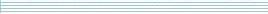| home      | people     | research     | publications    | seminars    | events     | contactCommunication Networks   | Systems Biology   | Hybrid Systems    | Machine Learning   | Dynamics & Interaction### Schools Mathematics Grand Challenge

Week five's Puzzles

Problem 9:

The ninth problem was:

The number 6 has the following property:

If you subtract two from it you get 4 which is a perfect square,

6 - 2 = 4 = (2 x 2) = 2^2

and if you add two to it you get 8 which is a perfect cube,

6 + 2 = 8 = (2 x 2 x 2) = 2^3.

What is the next whole number, strictly bigger than 6, with this property? (meaning that when you subtract two from it, you get a perfect square and when you add two to it, you get a perfect cube)

The solution was:

Let X be the number we seek. Then X + 2 is a perfect cube and X - 2 is a perfect square. One way to find the number we are looking for is to find the next cube number (after 8) such that when we subtract four from it we get a perfect square.

3^3 - 4 = 27 - 4 = 23 which is not a square
4^3 - 4 = 64 - 4 = 60 which is not a square
5^3 - 4 = 125 - 4 = 121 which is eleven squared.

So the answer is 125 - 2 = 123.

Problem 10:

The tenth problem was:

The factorial of a positive whole number n is written n! and is calculated by multiplying together all of the whole numbers from n back to one. For instance:

4! = 4x3x2x1 = 24
5! = 5x4x3x2x1 = 120
3! = 3x2x1 = 6
7! = 7x6x5x4x3x2x1 = 5040.

If you add up the factorials of all the whole numbers from 1 up to 1,000,000 (1 million) and divide the sum by 40, what will the remainder be?

So in this question we are asking for the remainder when you divide 1! + 2! + 3! + 4! + 5! + ... + 1,000,000! (the sum of the factorials of the first million positive whole numbers) by 40?

Remember that your answer must be a whole number between 0 and 39.

HINT: You do not need to work out the whole sum. Also be aware that calculators do not always give the right answer when you work with very large numbers!

The solution was:

The key point here is that if 40 divides evenly into n! for some positive number n, it will also divide evenly into the factorials of all numbers greater than n.

The first factorial into which 40 divides evenly is 5! = 5 x 4 x 3 x 2 x 1 = 120 = 40 x 3. Hence 40 divides evenly into

5! + 6! + 7! + ... + (1,000,000)!

and the remainder we seek is the same as the remainder when you divide 40 into

1! + 2! + 3! + 4! = 1 + 2 + 6 + 24 = 33.

So the answer is 33.